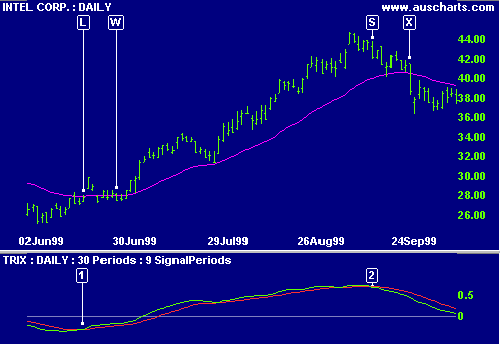# TRIX Indicator

TRIX is an oscillator designed for trading trends. Select a TRIX indicator period appropriate to the time frame that you are trading. The indicator will keep you in trends that are shorter or equal to the window period.

Based on a triple-smoothed moving average of Closing price, the indicator eliminates cycles shorter than the selected indicator period. Triple smoothing reduces volatility and minimizes the chance of false signals shaking you out of a trend too early.

TRIX was developed by Jack Huton, publisher of (Technical Analysis of) Stocks and Commodities magazine.

• Go long when TRIX turns up below zero.
• Go short when TRIX turns down above zero.

Use a signal line (a 9 day exponential moving average of TRIX) to eliminate false signals. Wait for TRIX to cross the signal line before entering the trade.

### Example

Intel Corporation is plotted with a      30-day exponential moving average (MA) and 30-day TRIX      indicator and      signal line.Mouse over chart captions to display trading signals.

Compare the results of the TRIX oscillator to those of a single moving average system using the MA with closing price as a filter:

1. Go long [L] when TRIX crosses to above the signal line while below zero.
Price closes above the MA 4 days earlier, but then whipsaws us in and out of several times at [W].
2. Go short [S] when TRIX crosses to below the signal line while above zero.
By comparison the MA signal [X] is much later.

It is evident that TRIX can eliminate a number of whipsaws, with only marginal lag in the signal compared to faster indicators.

### Setup

See Indicator Panel for directions on how to set up an indicator.

The default TRIX window is 12 days. To alter the default settings - Edit Indicator Settings.

## Formula

The Trix indicator is calculated as follows:

1. Decide on the number of periods (n) to include in the indicator window, based on the time frame that you are trading.
2. EMA1:                  Calculate an n-period exponential moving average of closing price
3. EMA2:                  Calculate an n-period exponential moving average of EMA1
4. EMA3:                  Calculate an n-period exponential moving average of EMA2
5. Calculate TRIX:    ( EMA3 [today] - EMA3 [yesterday] ) / EMA3 [yesterday]